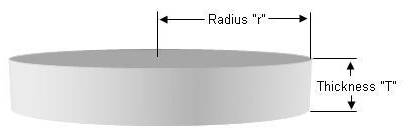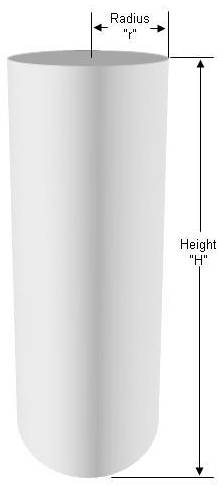# Free Concrete Calculator

Building your own concrete slab, patio driveway or walkway is an excellent home improvement and most diy home handymen are capable of completing this type of project. All that is required is some knowledge and the right tools.

### Concrete (Sonotube) or Column

Download a free automatic ready-mix, by the yard and quantities required of 60 lb. and 80 lb. pre-mixed bags - concrete calculator - (you must have Microsoft Excel or other compatible spreadsheet program)

Information on hand mixing concrete.

It should be noted that cement, is one of the 4 items that makes up concrete. Concrete is a mixture of cement, aggregate, sand and water.

### Square or Rectangle Concrete Slab:Notes:

• Pre-mix concrete comes in bags that are 60 lbs. and 80 lbs.
• A 60 lb. bag provides 0.45 cubic feet of cured concrete.
• A 80 lb. bag provides 0.6 cubic feet of cured concrete.
• If you have concrete delivered it is sold by the yard (which is a cubic yard = 27 cubic feet).

Following are the formulas to calculate how much concrete you will require for a square or rectangular shaped pour:

### Example:

A patio 10' long by 8' wide - 6″ thick

10 x 8 x 6 ÷ 12 = 40 cubic feet of concrete

10 x 8 x 6 ÷ 12 = 40 cubic feet of concrete

40 ÷ 0.6 = 67 (66.7) 80 lb. bags of concrete

40 ÷ 27 = 1.5 cubic yards of concrete

### Circular Concrete Slab:Notes:

• Pre-mix concrete comes in bags that are 60 lbs. and 80 lbs.
• A 60 lb. bag provides 0.45 cubic feet of cured concrete.
• A 80 lb. bag provides 0.6 cubic feet of cured concrete.
• If you have concrete delivered it is sold by the yard (which is a cubic yard = 27 cubic feet).

Following are the formulas to calculate how much concrete you will require for a circular slab:

### Example:

A circle 6″ thick where the circle has a radius of 5.5' (11' diameter circle)

3.14 x 5.5 x 5.5 x 6 ÷ 12 = 47.5 cubic feet of concrete

47.5 ÷ 0.45 = 106 (105.6) 60 lb bags of concrete

47.5 ÷ 0.6 = 80 (79.2) 80 lb. bags of concrete

47.5 ÷ 27 = 1.8 cubic yards of concrete

### Concrete Sonotube , Column Or Round Footing:Notes:

• Pre-mix concrete comes in bags that are 60 lbs. and 80 lbs.
• A 60 lb. bag provides 0.45 cubic feet of cured concrete.
• A 80 lb. bag provides 0.6 cubic feet of cured concrete.
• If you have concrete delivered it is sold by the yard (which is a cubic yard = 27 cubic feet).

Following are the formulas to calculate how much concrete you will require for a circle, tube or column shaped pour:

### Example:

A column 8' high where the column has a radius of 5″ (10″ diameter tube)

3.14 x 5 x 5 x 96 ÷ 1728 = 4.4 cubic feet of concrete

4.4 ÷ 0.45 = 10 (9.8) 60 lb bags of concrete

4.4 ÷ 0.6 = 8 (7.3) 80 lb. bags of concrete

4.4 ÷ 27 = 0.16 cubic yards of concrete